# Maths - Cubical Type Theory

### Spaces As Types

 As discussed on the page here HoTT relates types to spaces: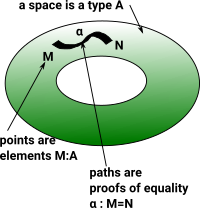On the page here is a discussion of proofs and how points can be considered equal if they have the same properties.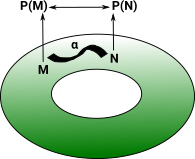There is a problem with modeling spaces in this way in the consistency of higher order equalities. To maintain consistency we need to model in a a less direct way by using the map out of an interval.### Interval

 An interval space II is a space with two points and a path between them 0 -> 1

Interval Eliminator

 The function coe defines dependent eliminator for I, it says that in order to define f : \Pi (i : I) -> P i for some P : I -> \Type it is enough to specify f left ```\func coe (P : I -> \Type) (a : P left) (i : I) : P i \elim i | left => a```

### Presheaf Categories

Cubical sets are presheaf categories:

Cubeop -> set

We need cubical because products are closed. This gives 'tiny' interval II in the presheaf category.

### Definition

Every type needs to define

• composition operators: hcomp
• coertion operators: transp

### Comparison to Proof in Martin-Löf Theory

 On the page here we looked at proofs in a more traditional Martin-Löf theory. When implemented (modeled) in Idris it is shown as an equality which we understand as a proposition. `(plus x Z) = x` To prove that this proposition is true we need to construct it like this: `Refl` In order to use cubical type theory as implemented (modeled) in 'cubicaltt' we need some changes. Instead of '=' it uses Path. `Path nat (add zero n) n` This path is constructed as a function from the interval like this: ` zero`
 So here is a proof in Idris using traditional Martin-Löf theory. ```total plusZeroRightNeutral : (x : Nat) -> (plus x Z) = x plusZeroRightNeutral Z = Refl plusZeroRightNeutral (S n) = rewrite (plusZeroRightNeutral n) in Refl``` Here is the same proof from 'cubicaltt'. Instead of '=' it uses Path. ```add_zero : (n : nat) -> Path nat (add zero n) n = split zero -> zero suc n -> suc (add_zero n @ i)```
 Where: PathP is a heterogeneous path type and is a builtin keyword. Path is a homogeneous path type and is defined here as a map from the interval to 'a'. So, instead of modeling '=' directly, it is instead modeled indirectly as 'Path'. ```-- Identity types Path (A : U) (a0 a1 : A) : U = PathP ( A) a0 a1 refl (A : U) (a : A) : Path A a a = a```

The interval <i> behaves like a type in some ways but not in others. See below for further discusion of the interval where it will be denoted as.

The first way of modeling proofs assumes that things can only be equal in one way but here we change this so that things can be equal in multiple different ways. The above example is comparing values so they can only be equal in one way. Below we compare bool to bool, this can be equal to itself in 2 ways:

• where true=true and false=false
• or where true=false and false=true
 Here (from this site) the second type of equality is constructed . Transporting true along this equality gives false and false gives true. isoToEquiv : type of A, type of B, A->B,B->A,y->path B,x->path A notK is a proof that not not b = b ```notEquiv : equiv bool bool = (not,isoToEquiv bool bool not not notK notK)``` We can glue these equalities together. ```-- Construct an equality in the universe using Glue notEq : Path U bool bool = Glue bool [ (i = 0) -> (bool,notEquiv) , (i = 1) -> (bool,idEquiv bool) ]```
 There are various related mathematical structures that give us multiple perspectives on this.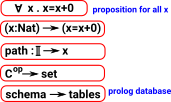### Contractible Space

A contractible space is one that can be continuously shrunk to a point within that space.

topological space X is contractible if:

• the identity map on X is null-homotopic,
• if it is homotopic to some constant map.

the following are all equivalent:

• X is contractible (i.e. the identity map is null-homotopic).
• X is homotopy equivalent to a one-point space.
• X deformation retracts onto a point. (However, there exist contractible spaces which do not strongly deformation retract to a point.)
• For any space Y, any two maps f,g: Y -> X are homotopic.
• For any space Y, any map f: Y -> X is null-homotopic.
 (from this site) ```-- The fiber/preimage of a map: fiber (A B : U) (f : A -> B) (y : B) : U = (x : A) * Path B y (f x) -- A map is an equivalence if its fibers are contractible isEquiv (A B : U) (f : A -> B) : U = (y : B) -> isContr (fiber A B f y)```

### Variables

When using 'logic' notation we often specify variables in a context to the left of a 'turnstyle' symbol.
 Γ,n:Nat(n=n+0)
In programming languages we might specify variables separately somewhere in scope.
```Nat n
n -> (n = n+0)```

When using cubical type theory we can specify variables in the formal interval:

If 'C' is the context, containing the variables, then Cop is the formal interval and a path is a presheaf Cop->Set.

```-- Identity types

Path (A : U) (a0 a1 : A) : U = PathP (<i> A) a0 a1

refl (A : U) (a : A) : Path A a a =<i> a```

### The Formal Interval

HoTT uses an idea from topology, the interval.represents the unit interval [0,1]

not totally ordered like classic interval.is like a type but it is not a type. Instead a path is a primitive notion in HoTT.

 used for variables i... with grammar r,s ::= 0 | 1 | i | 1-r | r/\s | r\/s where: 0 is least element 0:(1->) 1 is greatest element 1:(1->) r,s are variables r/\s is meet representing min /\:(->->) r\/s is join representing max \/:(->->) 1-r is an involution (its own inverse) where: 1-0=1 1-1=0 1-(r\/s) = (1-r)/\(1-s) 1-(r/\s) = (1-r)\/(1-s) (1-r):(->)### Paths

 PathAx y behaves like a function out of the interval p:-> A where: p 0 = x p 1 = y Althoughis not a proper type so we can't have a function into it for example.Transport turns path into equivalence.

## Glue

Glue types give us univalance.

Glue types turn an equivalence of types into a path:

ua: (A B : U) -> A ~ B -> PathU A B

#### Theory

 If we have a type B and a partial type A that is equivalent to B where it is defined then we can glue them together to form a new type.#### Example

 Here is an example of a binary type with two elements '0' and '1'. This type can be 'equal' to inself in two ways: 0=0 and 1=1 or, 0=1 and 1=0So we get two squares that must commute.

Gluing the top mappings together then give one square and the centre of the square can be filled in representing the isomorphism/equality of the whole type with itself.

 Glue type from Agda cubical here ```Glue :{L L'} (A : Set L) {φ : I} -> Partial φ (Σ[TSet L'] T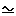A) -> Set L'``` constructor ```glue :{ L L'} {A : Set L} {φ : I} {Te : Partial φ ( Σ[TSet L'] TA)} -> PartialP φ T -> A -> Glue A Te``` eliminator ```unglue :{ L L'} {A : Set L} (φ : I) {Te : Partial φ (Σ[TSet L' ] TA)} -> Glue A Te -> A``` Where: Partial - allows Agda to define partially specified n-dimensional cubes. Setω - allows Agda to make sure everything has a 'type' in the presence of universe polymorphism. `Partial :{L} -> I -> Set L -> Setω`

### Composition of Paths and Transport

These may need to be defined for each type. This is done using the glue construct above.

 For traditional equalities and equivalences we have a relatively simple concept of transitivity to combine them. So in the case illustrated here: A=B B=C so we can deduce A=C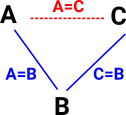This could be extended to multiple equalities which might be illustrated by a diagram like this where the missing face of a polygon can be inferred. This is related to a Horn clause discussed on page here.However, when we move to homotopy type equalities things get more complicated, we need to make sure all possible paths between the endpoints are included. See the discussion of composition of paths on page here.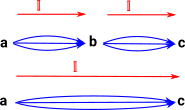### Example - List

 We have already looks at the 'bool' example. Here is slightly more complicated so that we can think about composition. So imagine a type which is a list containg the elements: 'x', 'y' and 'z'. If we have another list with these elements, but in a different order, then this would be isomorphic (but not equal) to the original list.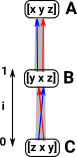## Presheaf

A 'presheaf' category is a special case of a functor category (see page here). It is a contravarient functor from a category 'C' to Set.

Since it is contravarient it is usually written:

Cop->Set

or

SetCop

There is more about presheaves on the page here.

### Presheaf Example - Single Element Set

A very simple example would be where Cop is a single element set (terminal object in set).

Hom( Cop, Set) therefore contains set of single arrows, one for every element of the set.### Presheaf Example - Graph

Here Cop is a category with two objects E (for edge) and V (for vertex) also two arrows s (for source) and t (for target).

This allows us to build a structure on top of set where the diagram on the right commutes.

We can therefore build up complex graphs from individual vertices and edges.### Presheaf - Interval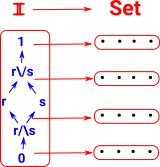This diagram tries to relate this to cubical complex bases on combinatorial notation as described on page here.A slightly more complex complex:### Combining intervals

 MeetJoin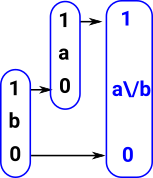### Modeling Algebra

 I'm trying to put this all together in one diagram - not correct yet.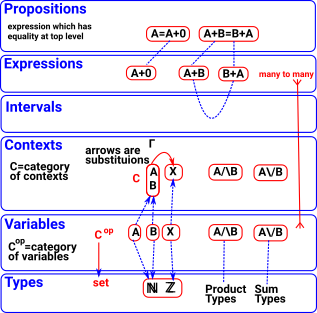#### Unification

The process of finding a substitution that makes two atomic sentences identical.

### Comparison with Intentional Type Theory

In M-L intentional type theory a proposition is either proven or not, if it was not then it may, or may not, be true. Since proofs in HoTT are canonical then we have other possibilities, it may be true, false, neither or both, also it may be true in many ways. These values represent truth values of propositions which correspond to paths in a space. This situation with different truth values is related to the idea of topos in category theory.

ITT HoTT

Proposition

a=b

Path

I->A
may still use a=b

Proof of that proposition

Refl: a=a

λi -> x

### Free De Morgan Algebra

De Morgan algebra is a generalisation of boolean algebra without rule of excluded middle.

#### Recap

So if we have 2 points 'n' and 'n+0' are they on a path/surface? This corresponds to the proposition 'n=n+0'. If we we using Boolean logic this proposition would be either true or false. In M-L type theory/constructive logic the proposition is either proven or its not, if its not then we can't say anything about it. Here the truth value is a free De Morgan algebra.### Fibrations

 A cubical complex might be thought of as a family of types where the cells are put into are hierarchy of types according to their dimension. These dimensions (and therefore types) are related by their boundary maps,### Presheaves

Presheaves allow us to build a more complex object out of simpler objects.

If C is a category of primitive objects then the presheaf is:

F: Cop -> Set

Objects of C Morphism in C
X
f: X -> Y
where:
F(X) is a set representing the ways X occurs inside F.

where:
F(f) : F(Y) -> F(X) is the function mapping each occurrence y of Y in F to the corresponding suboccurrence x (included in y through f) of X in F

 see nlab siteCubical sets are presheaves

Sheaves are discussed on page here.

### Kan Composition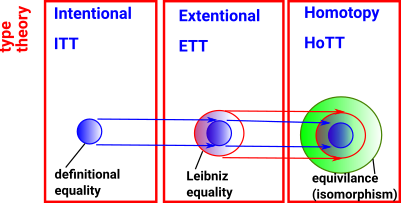## Implementations

 Agda CubicalTT Arend

Where I can, I have put links to Amazon for books that are relevant to the subject, click on the appropriate country flag to get more details of the book or to buy it from them.Computation and Reasoning - This book is about type theory. Although it is very technical it is aimed at computer scientists, so it has more discussion than a book aimed at pure mathematicians. It is especially useful for the coverage of dependant types.